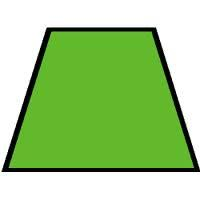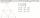# IS trapezoid

Isosceles trapezoid arm measured 35 cm. Height is 30 cm and middle segment is 65 cm. Determine length of its bases.

a =  83.03 cm
c =  46.97 cm

### Step-by-step explanation:Did you find an error or inaccuracy? Feel free to write us. Thank you!Tips to related online calculators
Do you have a linear equation or system of equations and looking for its solution? Or do you have a quadratic equation?

#### You need to know the following knowledge to solve this word math problem:

We encourage you to watch this tutorial video on this math problem:

## Related math problems and questions:

• IS trapezoidCalculate the length of diagonal u and height v of isosceles trapezoid ABCD, whose bases have lengths a = |AB| = 37 cm, c = |CD| = 29 cm and legs b = d = |BC| = |AD| = 28 cm.
• Medians in triangleMedian of isosceles triangle has a length 3 cm. Determine the length of its sides if its perimeter is 16 cm.
• Isosceles trapezoidFind the area of an isosceles trapezoid, if the bases are 12 cm and 20 cm, the length of the arm is 16 cm
• TrapezoidsIn the isosceles trapezoid ABCD we know: AB||CD, |CD| = c = 8 cm, height h = 7 cm, |∠CAB| = 35°. Find the area of the trapezoid.
• Isosceles trapezoidCalculate the circumference and the contents of the isosceles trapezoid if you know the size of the bases is 8 and 12 cm and the size of the arms is 5 cm.
• EstateAn estate-shaped rectangular trapezoid has bases long 34 m, 63 m, and perpendicular arm 37 m. Calculate how long its fence is.
• ISO trapezoid v2bases of Isosceles trapezoid measured 20 cm and 4 cm and its perimeter is 55 cm. What is the are of a trapezoid?
• ArmCalculate the length of the arm r of isosceles triangle ABC, with base |AB| = 14 cm and a height v=18 cm.
• Area of iso-trapFind the area of an isosceles trapezoid if the lengths of its bases are 16 cm and 30 cm, and the diagonals are perpendicular to each other.
• Isosceles trapezoidThe lengths of the bases of the isosceles trapezoid are in the ratio 5:3, the arms have a length of 5 cm and height = 4.8 cm. Calculate the circumference and area of a trapezoid.
• Isosceles trapezoidCalculate the area of an isosceles trapezoid whose bases are in the ratio of 4:3; leg b = 13 cm and height = 12 cm.
• TrapeziumThe area of trapezium is 35 cm2. Find its altitude if the bases are 6cm and 8 cm.
• Isosceles trapezoidCalculate the content of an isosceles trapezoid whose bases are at ratio 5:3, the arm is 6cm long and it is 4cm high.
• Isosceles + prismCalculate the volume of the perpendicular prism if its height is 17.5 cm and the base is an isosceles triangle with a base length of 5.8 cm and an arm length of 3.7 cm
• Isosceles trapezoidWhat is the height of an isosceles trapezoid, the base of which has a length of 11 cm and 8 cm and whose legs measure 2.5 cm?
• Arm and baseThe isosceles triangle has a circumference of 46 cm. Calculate its area if the arm is 5 cm longer than the base.
• RectangleThe width of the rectangle is 65% of its length. Perimeter of the rectangle is 132 cm. Determine the dimensions of the rectangle.